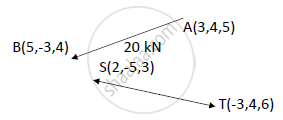# A Force of Magnitude of 20kn, Acts at Point A(3,4,5)M and Has Its Line of Action Passing Through B(5,-3,4)M. Calculate the Moment of this Force About a Line Passing Through Points S(2,-5,3) M and - Engineering Mechanics

A force of magnitude of 20kN, acts at point A(3,4,5)m and has its line of action passing through B(5,-3,4)m. Calculate the moment of this force about a line passing through points S(2,-5,3) m and T(-3,4,6)m.

#### Solutionoverline(F1) = 20 [((5-3)i +(-3-4)j+(4-5)k)/(sqrt(((5-3)^2+(-3-4)^2)+(4-5)^2))] =5.44i- 19.05j -2.72 k  kN

overlineM_(SF)1 = overline(SA) xx overline(F1) =

[[i,j,k],[3-2,4-(-5),5-3],[5.44,-19.05,-2.72]]  =13.62i+13.6j -68.01 k  kN-m

|M_(s^(F1))| = sqrt((13.62)^2 +(-13.6)^2+(-68.01)^2)=70.68 kN-m

hat(ST)= (overline(ST))/|overline(ST)|  ((-3-2)i+(4+5)j+(6-3)k)/(sqrt((-3-2)^2+(4+5)^2+(6-3)^2)) = -0.466i+0.839j +0.28k

M_(ST^(F1)) = M_(S^(F1)). hat(ST) =(13.62 i + 13.6 j – 68.01 k).(-0.466 i + 0.839 j + 0.28 k)
= -6.35 + 11.41 – 19.04

M_(ST^(F1) = -13.98 kN-m

Vector form,

overlineM_(ST^ (F1)) = M_(ST^ (F1)). hat(ST) = -13.98(-0.466 i + 0.839 j + 0.28 k)

M_(ST^(F1)) = 6.51 i – 11.73 j – 3.91 k

The moment of the force about a line passing through points S(2,-5,3) m and T(-3,4,6)m is -13.98 kN-m (magnitude) and 6.51 i – 11.73 j – 3.91 k (vector form).

Concept: Moment of force about a point
Is there an error in this question or solution?